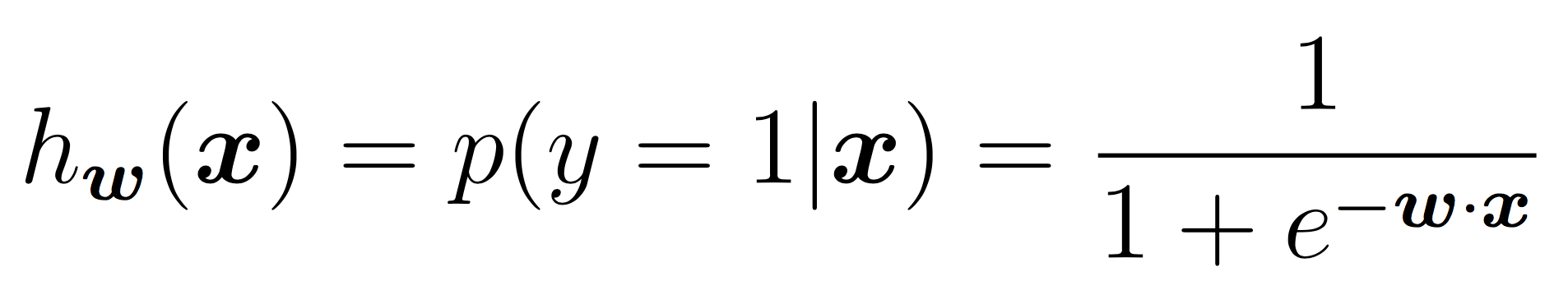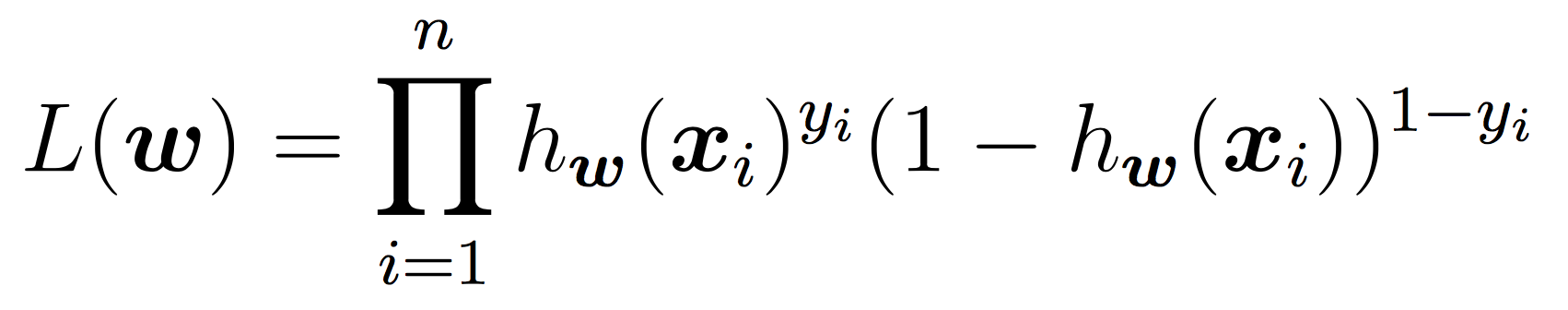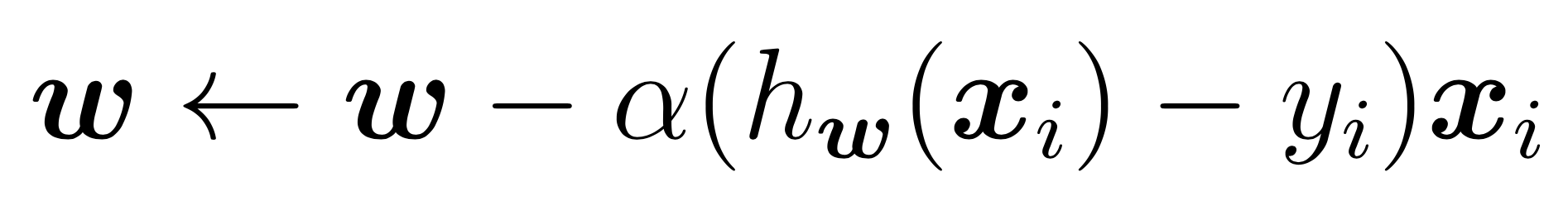CS360 Lab 5: Logistic Regression

Overview

The goals of this week’s lab:

• Understand and implement Logistic Regression
• Apply Logistic Regression to a real-world dataset

In this lab we will be analyzing a phoneme dataset:

• The task of phoneme classification is to predict the label of a phoneme articulation given its corresponding voice signal. In this problem, we have voice signals from two different phonemes: “aa” (as in “bot”) and “ae” (as in “bat”). They are from an acoustic-phonetic corpus called TIMIT. Phoneme classification is an important part of a speech-to-text system.

Partner work

Before getting started on the lab, find your random partner. You have two options for this part (or you can do both!) The code review can be quick, so it would be ideal to do both.

1. CODE REVIEW For this part, each person should choose a piece of code (a single file is ideal) that they have written in the past (must be in Python, ideally from this class Labs 1-4). The purpose of the code review is to develop better style and readability, and it is a common practice in industry and academia. After pulling up your code, switch monitors and go through your partner’s code. Can you understand what is going on? Then write down a few quick notes:
• Highlight one strength of the code.

• Highlight one area for improvement (long lines, poor variable/function name choices, not enough line breaks, functions that are too long or two short, confusing flow of functions, confusing main, lack of TDD, no comments, no function/method headers, poor use of capitals, etc).

After writing these down, share your feedback with your partner (and visa versa). Feel free to ask me questions if you’re unsure what is proper Python style. Or consult the Python style guide.

1. HANDOUT 11 Alternatively/additionally, go through any part of Handout 11 that we didn’t get to in class (or that remains confusing). These are good practice questions for making sure you understand logistic regression before you implement it.

Getting Started

Find your git repo for this lab assignment the lab05 directory. You should have the following files:

• input - directory containing train/test data sets. You may add additional data sets here.
• run_LR.py - your main program executable for Logistic Regression.
• LogisticRegression.py - file for the LogisticRegression class (and/or functions).
• README.md - for analysis questions and lab feedback.

Usage and I/O

Usage

Your programs should take in the same command-line arguments as Lab 4 (feel free to reuse the argument parsing code), plus a parameter for the learning rate alpha. For example, to run Logistic Regression on the phoneme example:

python3 run_LR.py -r input/phoneme_train.csv -e input/phoneme_test.csv -a 0.02

Program Inputs

To simplify preprocessing, you may assume the following:

• The datasets will have continuous features, and binary labels (0, 1). The train and test datasets will be in CSV form (comma separated values), with the label as the last column.

• I would recommend creating a function to parse the CSV file format. Use the method split(',') to split the line (type string) into a list of strings, which can be parsed further.

• Make sure the user enters a positive alpha value (it should be type float)

Program Outputs

• Your program should print a confusion matrix and accuracy of the result (see examples below).

• The design of your solution is largely up to you. You don’t necessarily need to have a class, but you should use good top-down design principles so that your code is readable. Having functions for the cost, for SGD, for the logistic function, etc is a good idea.

Logistic Regression

You will implement the logistic regression task discussed in class for binary classification.

Model

For now you should assume that the features will be continuous and the response will be a discrete, binary output. In the case of binary labels, the probability of a positive prediction is:To model the intercept term, we will include a bias term. In practice, this is equivalent to adding a feature that is always “on”. For each instance in training and testing, append a 1 to the feature vector. Ensure that your logistic regression model has a weight for this feature that it can now learn in the same way it learns all other weights.

Training

To learn the weights, you will apply stochastic gradient descent as discussed in class until the cost function does not change in value (very much) for a given iteration. As a reminder, our cost function is the negative log of the likelihood function, which is:Our goal is to minimize the cost using SGD. We will use the same idea as in Lab 3 for linear regression. Pseudocode:

initialize weights to 0's
while not converged:
shuffle the training examples
for each training example xi:
calculate derivative of cost with respect to xi
weights = weights - alpha*derivative
compute and store current cost

The SGD updates for w term are:The hyper-parameter alpha (learning rate) should be sent as a parameter to your SGD and used in training. A few notes for the above:

• In Lab 3 we did not actually shuffle the data points to make gradient descent truly “stochastic”, but for this lab you should (also make sure y is shuffled along with X!). You may use:
numpy.random.shuffle(A)

which by default shuffles along the first axis.

• The stopping criteria should be a) a maximum of some number of iterations (your choice) OR b) the cost has changed by less some number between two iterations (your choice).

• Many of the operations above are on vectors. I recommend using numpy features such as dot product to make the code simple.

• Try to choose hyper-parameters that maximize the testing accuracy.

Prediction

For binary prediction, apply the equation:and output the more likely binary outcome. You may use the Python math.exp function to make the calculation in the denominator. Alternatively, you may use the weights directly as a decision boundary as discussed in class.

The choice of hyper-parameters will affect the results, but you should be able to obtain at least 90% accuracy on the testing data. Make sure to print a confusion matrix and output a file of predicted labels.

Example Output

Here is an example run for testing purposes. You should be able to get a better result than this, but this will check if your algorithm is working. The details are:

• No shuffling of the training data points (which you should do for the final result!)
• alpha = 0.02
• stopping criteria: looking for changes in the cost J(w)
• epsilon = 1e-4 (see if cost has changed by this amount)
\$ python3 run_LR.py -r input/phoneme_train.csv -e input/phoneme_test.csv -a 0.02
Accuracy: 0.875000 (70 out of 80 correct)

prediction
0  1
------
0| 36  4
1|  6 34

Analysis and Comparison

1. So far, we have run logistic regression and Naive Bayes on different types of datasets. Why is that? Discuss the types of features and labels that we worked with in each case.

2. Explain how you could transform the CSV dataset with continuous features to work for Naive Bayes. You could use something similar to what we did for decision trees, but try to think about alternatives.

3. Then explain how you could transform the ARFF datasets with discrete features to work with logistic regression. What issues need to be overcome to apply logistic regression to the zoo dataset?

Extensions (optional)

Please include a discussion of your extension in your README. If this week’s lab was quick for you, I strongly recommend thinking about implementing softmax regression (logistic regression extended to the multi-class setting).

1. Implement one of your suggestions from your README so that you can properly compare these two algorithms. One concrete suggestion: either create a method that will convert ARFF to CSV, or visa versa. You will have to transform some of the features (and possibly outputs) along the way, but that way you won’t have to modify your algorithms directly.

For making logistic regression work in the multi-class setting, you have two options. You can use the likelihood function presented in class and run SGD. Or you can create K separate binary classifiers that separate the data into class k and all the other classes. Then choose the prediction with the highest probability.

1. So far we have not included regularization. Add an optional parameter lambda and explore adding regularization to the cost function and SGD. Did this improve the results? Did it improve the runtime?